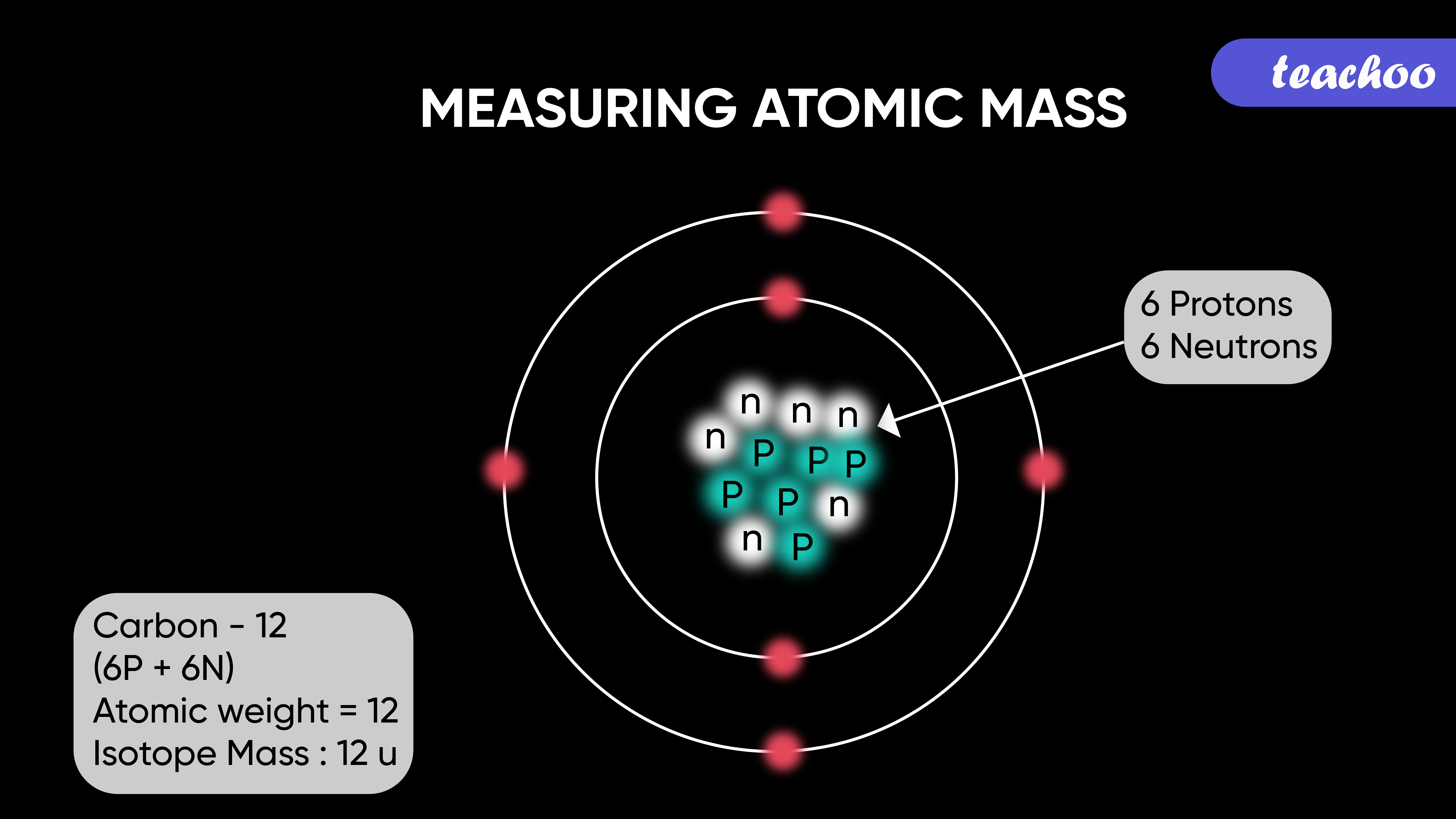According to Dalton, each element had a characteristic mass called atomic mass

It is denoted by amu (atomic mass unit) or simply u.

According to the International System of Units, the unit of atomic mass is u (unified mass)

## Drawbacks of Atomic Theory

We know that atoms are very tiny particles, hence it was very difficult to accurately measure their mass.

Hence scientists started using relative masses to measure the mass of different atoms.

For example,

In Carbon Monoxide (CO) 3g of Carbon reacts with 4g of Oxygen.

2C + O 2 →  2CO

12g    16g          28g

=  3g      4g           12g

Dividing throughout by 3;

=  3/3g    4/3g      12/3g

=  1g       4/3g        4g

Therefore, 1g of Carbon reacts with 4/3 g of Oxygen.

That is, Carbon requires 4/3 (=1.33) times more Oxygen in order to form CO.

These numbers were fractional and tedious, hence scientists had to come up with a more accurate method.

## Measuring atomic mass

So, scientists defined 1u = 1/16th mass of an Oxygen atom

1. It was chosen because Oxygen reacts with many atoms to form different compounds
2. It gave the atomic masses of most of the atoms as whole numbers## Drawbacks of Atomic Theory

The relative atomic masses of all elements have been found with respect to an atom of carbon-12 isotope.

Example -

In text Question - Page 35 Q1,Q2

## Atomic Mass of Different Elements

### Define Atomic Mass Unit

It is a unit of mass which is equal to mass of 1/12 of atom of Carbon -12 isotope

It is used to express and compare mass of different atoms and molecules

It is expressed in u (Atomic Mass Unit)

## Drawbacks of Atomic Theory

It is expressed in u (Atomic Mass Unit)

Example

• Atomic Mass Unit of Hydrogen = 1 u
• Atomic Mass Unit of Carbon = 12 u
• Atomic Mass Unit of Nitrogen = 14 u

Example -

In text Question -

NCERT Question -

1. Class 9
2. Chapter 3 Class 9 - Atoms And Molecules (Term 2)
3. Concepts

Concepts

Class 9
Chapter 3 Class 9 - Atoms And Molecules (Term 2)